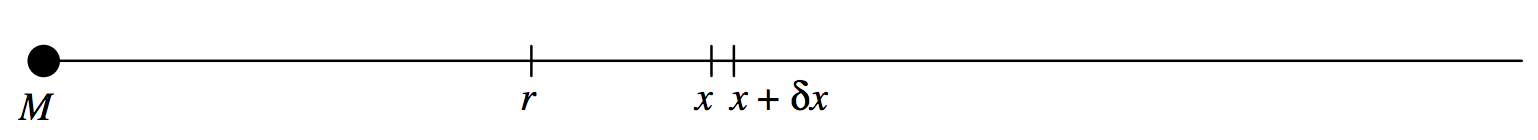$$\require{cancel}$$

# 5.8.1: Potential Near a Point Mass

•• Contributed by Jeremy Tatum
• Emeritus Professor (Physics & Astronomy) at University of Victoria

We shall define the potential to be zero at infinity. If we are in the vicinity of a point mass, we shall always have to do work in moving a test particle away from the mass. We shan’t reach zero potential until we are an infinite distance away. It follows that the potential at any finite distance from a point mass is negative. The potential at a point is the work required to move unit mass from infinity to the point; i.e., it is negative.$$\text{FIGURE V.23}$$

The magnitude of the field at a distance $$x$$ from a point mass $$M$$ (figure $$\text{V.23}$$) is $$GM/x^2$$, and the force on a mass m placed there would be $$GMm/x^2$$. The work required to move $$m$$ from $$x$$ to $$x + δx$$ is $$GMmδx/x^2$$ . The work required to move it from $$r$$ to infinity is

$GMm∫_r^∞ \frac{dx}{x^2} = \frac{GMm}{r}.$

The work required to move unit mass from $$∞$$ to $$r$$, which is the potential at $$r$$ is

$ψ = -\frac{GM}{r}. \label{5.8.1} \tag{5.8.1}$

The mutual potential energy of two point masses a distance r apart, which is the work required to bring them to a distance $$r$$ from an infinite initial separation, is

$V = -\frac{GMm}{r}. \label{5.8.2} \tag{5.8.2}$

I here summarize a number of similar-looking formulas, although there is, of course, not the slightest possibility of confusing them. Here goes:

Force between two masses:

$F = \frac{GMm}{r^2}. \quad \text{N} \label{5.8.3} \tag{5.8.3}$

Field near a point mass:

$g = \frac{GM}{r^2}, \quad \text{N kg}^{-1} \ or \text{ m s}^{-2} \label{5.8.4} \tag{5.8.4}$

which can be written in vector form as:

$\textbf{g} = - \frac{GM}{r^2} \hat{\textbf{r}} \quad \text{N kg}^{-1} \ or \text{ m s}^{-2} \label{5.8.5} \tag{5.8.5}$

or as:

$\textbf{g} = -\frac{GM}{r^3} \textbf{r}. \quad \text{N kg}^{-1} \ or \text{ m s}^{-2} \label{5.8.6} \tag{5.8.6}$

Mutual potential energy of two masses:

$V = -\frac{GMm}{r}. \quad \text{J} \label{5.8.7} \tag{5.8.7}$

Potential near a point mass:

$ψ = -\frac{GM}{r}. \quad \text{J kg}^{-1} \label{5.8.8} \tag{5.8.8}$

I hope that’s crystal clear.